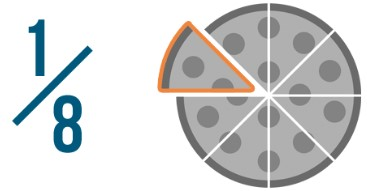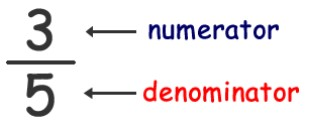# Fraction Calculator

Enter integers to make fractions and select the operation to solve fractions using fraction calculator.

Fraction Worksheets

Give Us Feedback

## Fraction Calculator

Fractions calculator takes the fractions from users and performs the following operations.

• Subtracting fractions
• Multiplying fractions
• Dividing fractions

Let’s dive into fractions by exploring fraction definition, how to simplify fractions without fraction simplifier, and how do you subtract, add, multiply, or divide fractions manually.

## What is a fraction?

Fractions are used to represent part of a whole. When we divide something in parts, a fraction shows the number of parts we have out of total quantity.

For example: If a pizza have total of 8 slices then 1 slice out of 8 can be expressed in fraction as 1/8.The upper part of fraction is known as numerator while the lower part of fraction is known as denominator.## How to simplify fractions?

This calculator for fractions or fraction solver performs all of the following operations.

Example: Perform addition, subtraction, multiplication and division on given fraction.

2/3 and 3/4

Step 1: Make the denominator of both fractions same.

= 2×4/3×4 + 3×3/4×3

= 8/12 + 9/12

Step 2: Add the numerator of both fractions and write the denominator by taking it common.

= (8 + 9)/12

= 17/12

2/3 + 3/4 = 17/12

By subtracting the numerators in the end, you can subtract fractions in the same way.

### How to multiply fractions?

Step 1: Multiply the numerator and denominators of both fractions to each other.

= 2/3 × 3/4

= (2×3)/(3×4)

= 6/12

= 1/2

### How to divide fractions?

Step 1: Reverse the second fraction by interchanging the position of numerator and denominator. Also replace the ÷ sign by × sign.

2/3 ÷ 3/4

2/3 × 4/3

Step 2: Now, multiply the numerator and denominators of both fractions to each other.

2/3 × 4/3 = 8/9

Add fractions calculator can accomplish all of the above performed operations on fractions.

### Other Languages